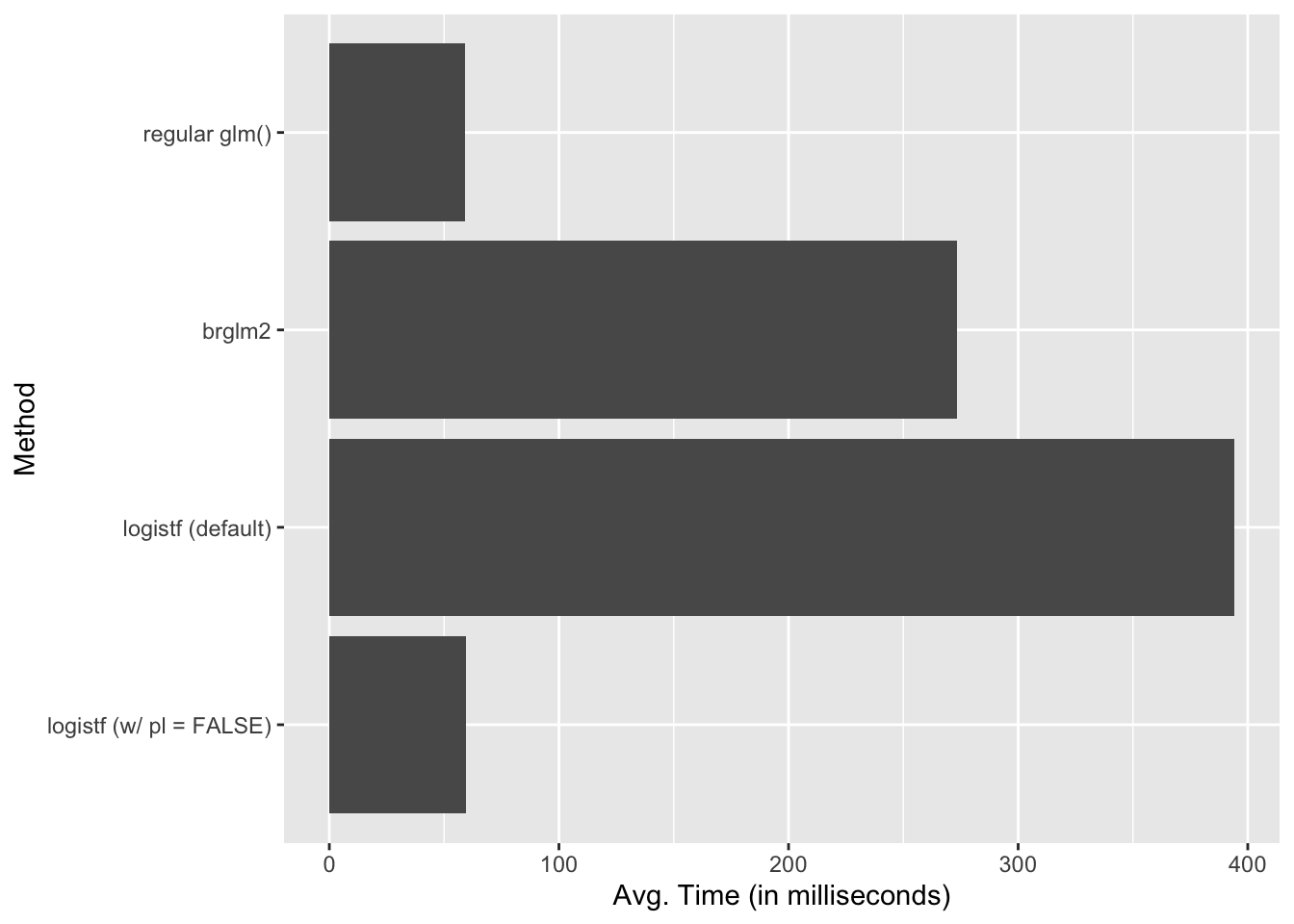# Benchmarking Firth’s Logit: {brglm2} versus {logistf}

logistf() is really fast

logistic regression
small samples
Firth
computing
R
In this post, I benchmark the brglm2 and logistf packages for fitting logistic regression models with Firth’s penalty.
Author

Carlisle Rainey

Published

August 11, 2023

## Firth’s Logit

I like Firth’s logistic regression model . I talk about that in Rainey and McCaskey (2021) and this Twitter thread. Kosmidis and Firth (2021) offer an excellent, recent follow-up as well.

I’ll refer you to the papers for a careful discussion of the benefits, but Firth’s penalty reduces the bias and variance of the logit coefficients.

## Goals for Benchmarking

In this post, I want to compare the brglm2 and logistf packages. Which fits logistic regression models with Firth’s penalty the fastest?

These packages both fit the models almost instantly, so there is no practical difference when fitting just one model. But large Monte Carlo simulations (or perhaps bootstraps), small differences might add up to a substantial time difference.

Here, I benchmark the two packages for fitting logistic regression models with Firth’s penalty in a small sample–the results might not generalize to a larger sample. The data set comes from Weisiger (2014) (see `?crdata::weisiger2014`). It has only 35 observations.

You can find the benchmarking code as a GitHub Gist.

## Benchmarking

I benchmark four methods here.

1. A vanilla `glm()` logit model.
2. A Firth’s logit via brglm2 by supplying `method = brglm2::brglmFit` to `glm()`.
3. A Firth’s logit via logistf via `logistf()` using the default settings.
4. A Firth’s logit via logistf via `logistf()` with the argument `pl = FALSE`. This argument is important because it skips hypothesis testing using profile likelihoods, which are computationally costly.
``````# install crdata package to egt weisiger2014 data set
remotes::install_github("carlislerainey/crdata")

library(tidyverse)
library(brglm2)
library(logistf)
library(microbenchmark)

weis <- crdata::weisiger2014

# rescale weisiger2014 explanatory variables using arm::rescale()
rs_weis <- weis %>%
mutate(across(polity_conq:coord, arm::rescale))

# create functions to fit models
f <- resist ~ polity_conq + lndist + terrain + soldperterr + gdppc2 + coord
f1 <- function() {
glm(f, data = rs_weis, family = "binomial")
}
f2 <- function() {
glm(f, data = rs_weis, family = "binomial", method = brglmFit)
}
f3 <- function() {
logistf(f, data = rs_weis)
}
f4 <- function() {
logistf(f, data = rs_weis, pl = FALSE)
}

# do benchmarking
bm <- microbenchmark("regular glm()" = f1(),
"brglm2" = f2(),
"logistf (default)" = f3(),
"logistf (w/ pl = FALSE)" = f4(),
times = 100) ``````
``````Warning in microbenchmark(`regular glm()` = f1(), brglm2 = f2(), `logistf
(default)` = f3(), : less accurate nanosecond times to avoid potential integer
overflows``````
``print(bm)``
``````Unit: microseconds
expr      min        lq      mean    median        uq
regular glm()  500.651  547.0425  591.5304  560.0805  584.4550
brglm2 2469.266 2546.9405 2735.6438 2591.5690 2672.3800
logistf (default) 3303.329 3432.6635 3942.9122 3481.6585 3558.1235
logistf (w/ pl = FALSE)  513.197  553.3155  594.6205  570.8225  608.9115
max neval
2713.708   100
9735.983   100
17672.148   100
1698.343   100``````

In short, logistf is slower than brglm2, but only because it computes the profile likelihood p-values by default. Once we skip those calculations using `pl = FALSE`, logistf is much faster. On average, it’s faster than `glm()`, because `glm()` has the occasional really slow computation.

Here’s a plot showing the computation times of the four fits. Remember that all of these are computed practically instantly, so it only makes a difference when the fits are done thousands of times, like in a Monte Carlo simulation.

``````# plot times
bm %>%
group_by(expr) %>%
summarize(avg_time = mean(time)*10e-5) %>%  # convert to milliseconds
ggplot(aes(x = fct_rev(expr), y = avg_time)) +
geom_col() +
labs(x = "Method",
y = "Avg. Time (in milliseconds)") +
coord_flip()``````# Follow-Up Notes

The models return slightly different estimates. Maybe they are using slightly different convergence tolerances. I didn’t investigate this beyond noticing it.

``cbind(coef(f2()), coef(f4()))``
``````                  [,1]       [,2]
(Intercept) -0.4771934 -0.4771935
polity_conq -2.2771109 -2.2771117
lndist       3.4020241  3.4020215
terrain      1.1018696  1.1018713
soldperterr -0.5952096 -0.5952110
gdppc2      -1.1542010 -1.1541998
coord        3.0514481  3.0514473``````

Ioannis Kosmidis made me aware of two things.

1. logistf has a C++ backend (thus explaining the speed).
2. brglm2 is written entirely in R. He noted that a new version is coming out soon that might be substantially faster. (brglm2 is also more general; it supports a variety of models and corrections).

## Computer

Here’s the info on my machine.

``system("sysctl -n machdep.cpu.brand_string", intern = TRUE)``
`` "Apple M2 Max"``

## References

Firth, David. 1993. “Bias Reduction of Maximum Likelihood Estimates.” Biometrika 80 (1): 27–38. https://doi.org/10.1093/biomet/80.1.27.
Kosmidis, Ioannis, and David Firth. 2021. “Jeffreys-Prior Penalty, Finiteness and Shrinkage in Binomial-Response Generalized Linear Models.” Biometrika 108 (1): 71–82. https://doi.org/10.1093/biomet/asaa052.
Rainey, Carlisle, and Kelly McCaskey. 2021. “Estimating Logit Models with Small Samples.” Political Science Research and Methods 9 (3): 549–64. https://doi.org/10.1017/psrm.2021.9.
Weisiger, Alex. 2014. “Victory Without Peace: Conquest, Insurgency, and War Termination.” Conflict Management and Peace Science 31 (4): 357–82. https://doi.org/10.1177/0738894213508691.